# Lecture-8.ppt

28 May 2023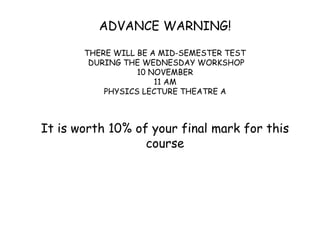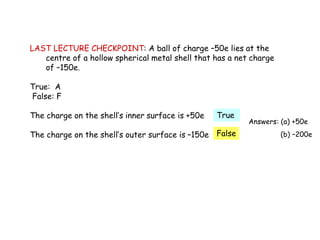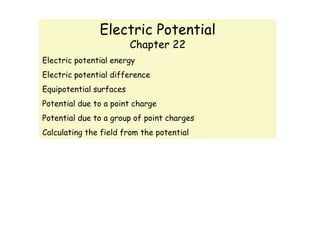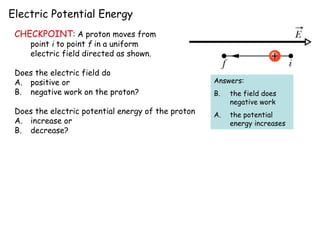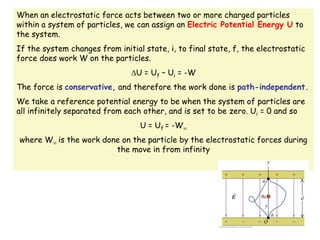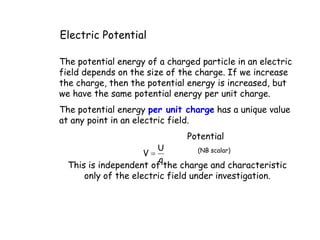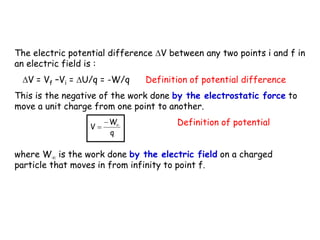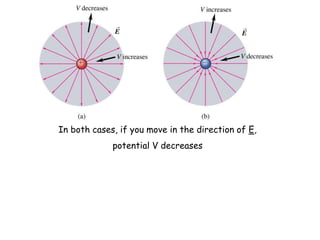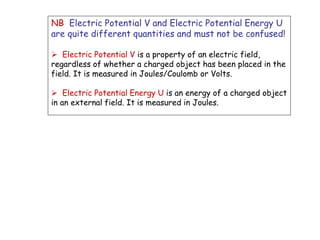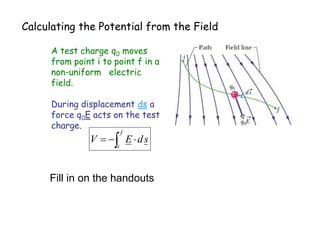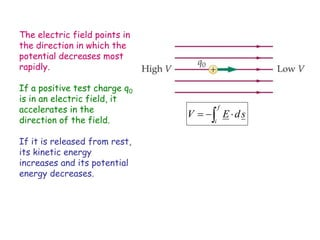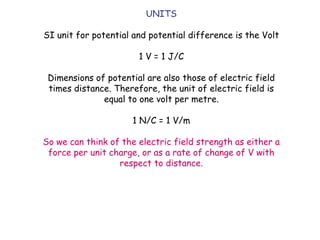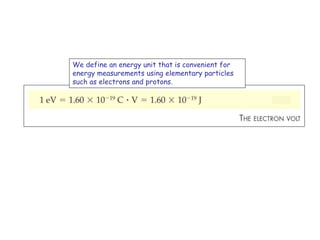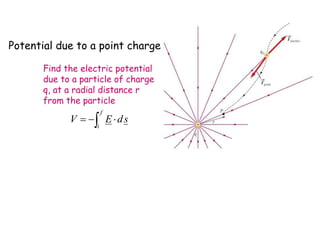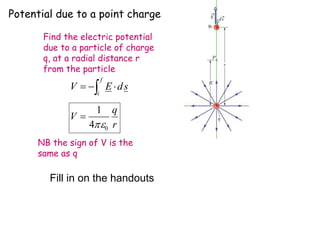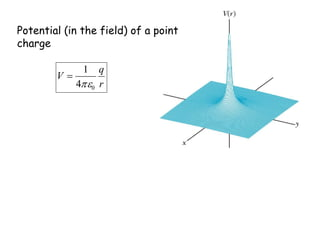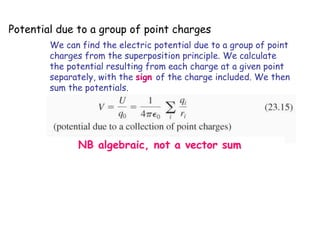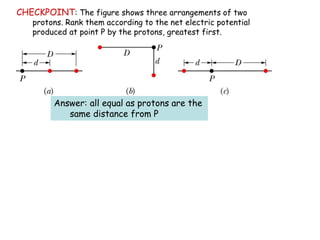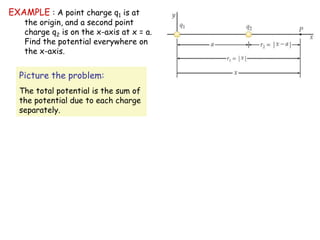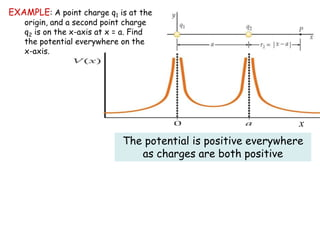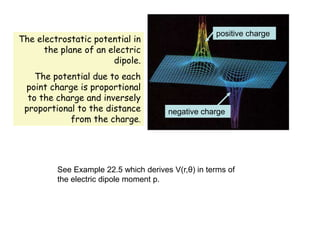1 sur 21

### Lecture-8.ppt

• 1. ADVANCE WARNING! THERE WILL BE A MID-SEMESTER TEST DURING THE WEDNESDAY WORKSHOP 10 NOVEMBER 11 AM PHYSICS LECTURE THEATRE A It is worth 10% of your final mark for this course
• 2. LAST LECTURE CHECKPOINT: A ball of charge –50e lies at the centre of a hollow spherical metal shell that has a net charge of –150e. True: A False: F The charge on the shell’s inner surface is +50e The charge on the shell’s outer surface is –150e Answers: (a) +50e (b) –200e True False
• 3. Electric Potential Chapter 22 Electric potential energy Electric potential difference Equipotential surfaces Potential due to a point charge Potential due to a group of point charges Calculating the field from the potential
• 4. Electric Potential Energy CHECKPOINT: A proton moves from point i to point f in a uniform electric field directed as shown. Does the electric field do A. positive or B. negative work on the proton? Does the electric potential energy of the proton A. increase or B. decrease? Answers: B. the field does negative work A. the potential energy increases
• 5. When an electrostatic force acts between two or more charged particles within a system of particles, we can assign an Electric Potential Energy U to the system. If the system changes from initial state, i, to final state, f, the electrostatic force does work W on the particles. U = Uf – Ui = -W The force is conservative, and therefore the work done is path-independent. We take a reference potential energy to be when the system of particles are all infinitely separated from each other, and is set to be zero. Ui = 0 and so U = Uf = -W where W is the work done on the particle by the electrostatic forces during the move in from infinity
• 6. Electric Potential The potential energy of a charged particle in an electric field depends on the size of the charge. If we increase the charge, then the potential energy is increased, but we have the same potential energy per unit charge. The potential energy per unit charge has a unique value at any point in an electric field. Potential (NB scalar) This is independent of the charge and characteristic only of the electric field under investigation. q U V 
• 7. The electric potential difference V between any two points i and f in an electric field is : V = Vf –Vi = U/q = -W/q Definition of potential difference This is the negative of the work done by the electrostatic force to move a unit charge from one point to another. Definition of potential where W is the work done by the electric field on a charged particle that moves in from infinity to point f. q W V   
• 8. In both cases, if you move in the direction of E, potential V decreases
• 9. NB Electric Potential V and Electric Potential Energy U are quite different quantities and must not be confused!  Electric Potential V is a property of an electric field, regardless of whether a charged object has been placed in the field. It is measured in Joules/Coulomb or Volts.  Electric Potential Energy U is an energy of a charged object in an external field. It is measured in Joules.
• 10. Calculating the Potential from the Field A test charge q0 moves from point i to point f in a non-uniform electric field. During displacement ds a force q0E acts on the test charge. s d E V f i     Fill in on the handouts
• 11. The electric field points in the direction in which the potential decreases most rapidly. If a positive test charge q0 is in an electric field, it accelerates in the direction of the field. If it is released from rest, its kinetic energy increases and its potential energy decreases. s d E V f i    
• 12. UNITS SI unit for potential and potential difference is the Volt 1 V = 1 J/C Dimensions of potential are also those of electric field times distance. Therefore, the unit of electric field is equal to one volt per metre. 1 N/C = 1 V/m So we can think of the electric field strength as either a force per unit charge, or as a rate of change of V with respect to distance.
• 13. We define an energy unit that is convenient for energy measurements using elementary particles such as electrons and protons.
• 14. Potential due to a point charge Find the electric potential due to a particle of charge q, at a radial distance r from the particle s d E V f i    
• 15. Potential due to a point charge Find the electric potential due to a particle of charge q, at a radial distance r from the particle s d E V f i     r q V 0 4 1   NB the sign of V is the same as q Fill in on the handouts
• 16. r q V 0 4 1   Potential (in the field) of a point charge
• 17. Potential due to a group of point charges We can find the electric potential due to a group of point charges from the superposition principle. We calculate the potential resulting from each charge at a given point separately, with the sign of the charge included. We then sum the potentials. NB algebraic, not a vector sum
• 18. CHECKPOINT: The figure shows three arrangements of two protons. Rank them according to the net electric potential produced at point P by the protons, greatest first. Answer: all equal as protons are the same distance from P
• 19. EXAMPLE : A point charge q1 is at the origin, and a second point charge q2 is on the x-axis at x = a. Find the potential everywhere on the x-axis. Picture the problem: The total potential is the sum of the potential due to each charge separately.
• 20. EXAMPLE: A point charge q1 is at the origin, and a second point charge q2 is on the x-axis at x = a. Find the potential everywhere on the x-axis. x The potential is positive everywhere as charges are both positive
• 21. The electrostatic potential in the plane of an electric dipole. The potential due to each point charge is proportional to the charge and inversely proportional to the distance from the charge. positive charge negative charge See Example 22.5 which derives V(r,θ) in terms of the electric dipole moment p.